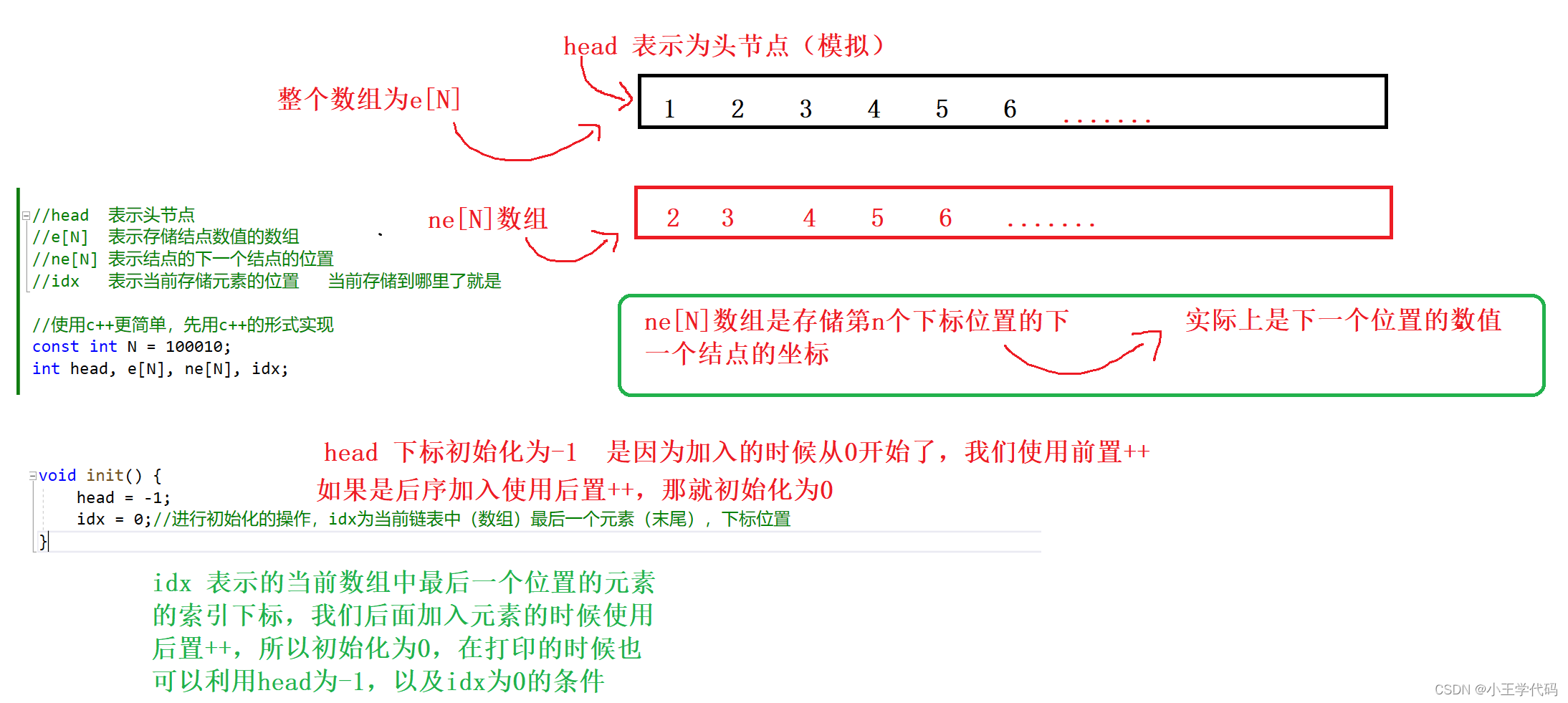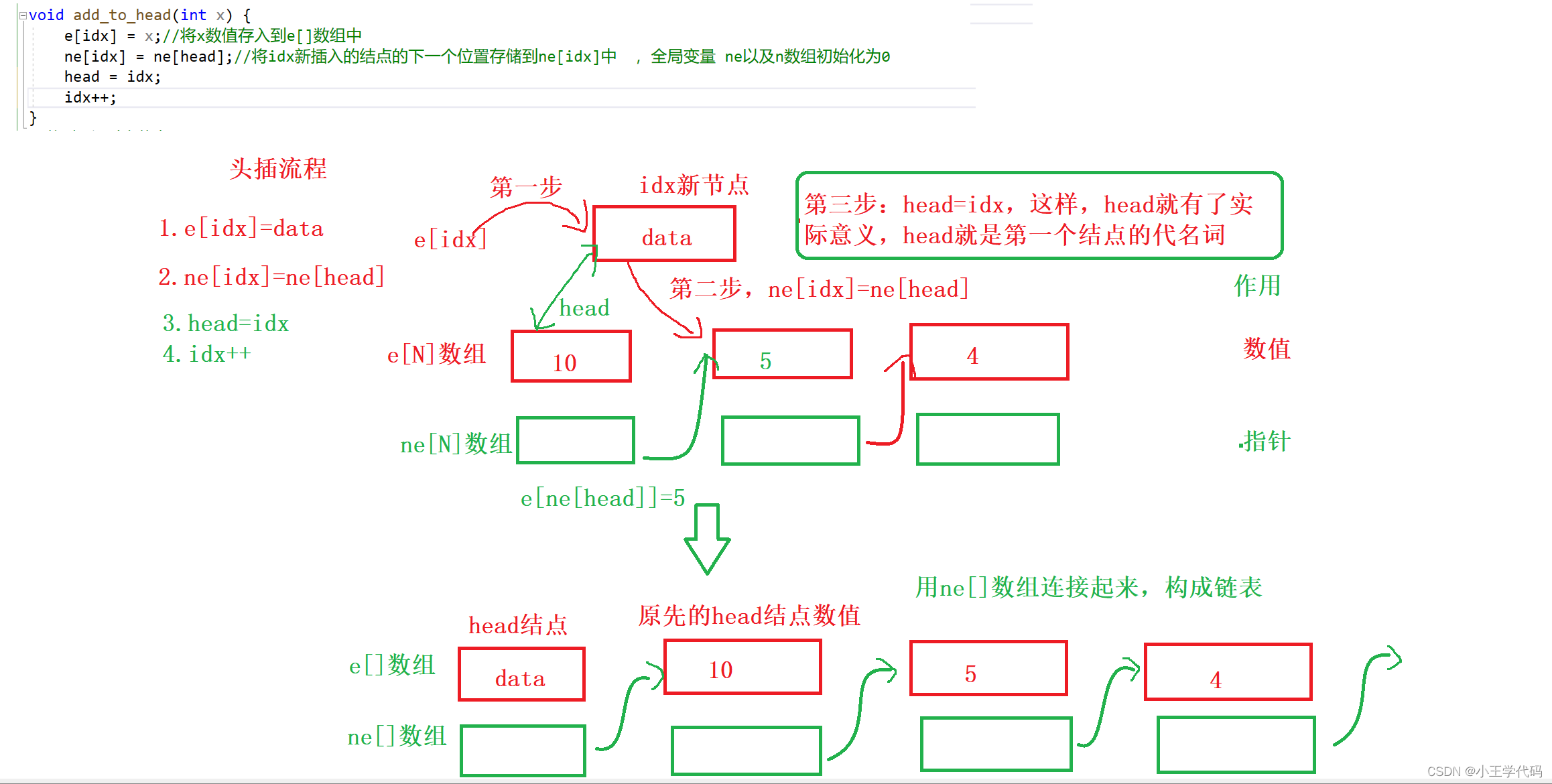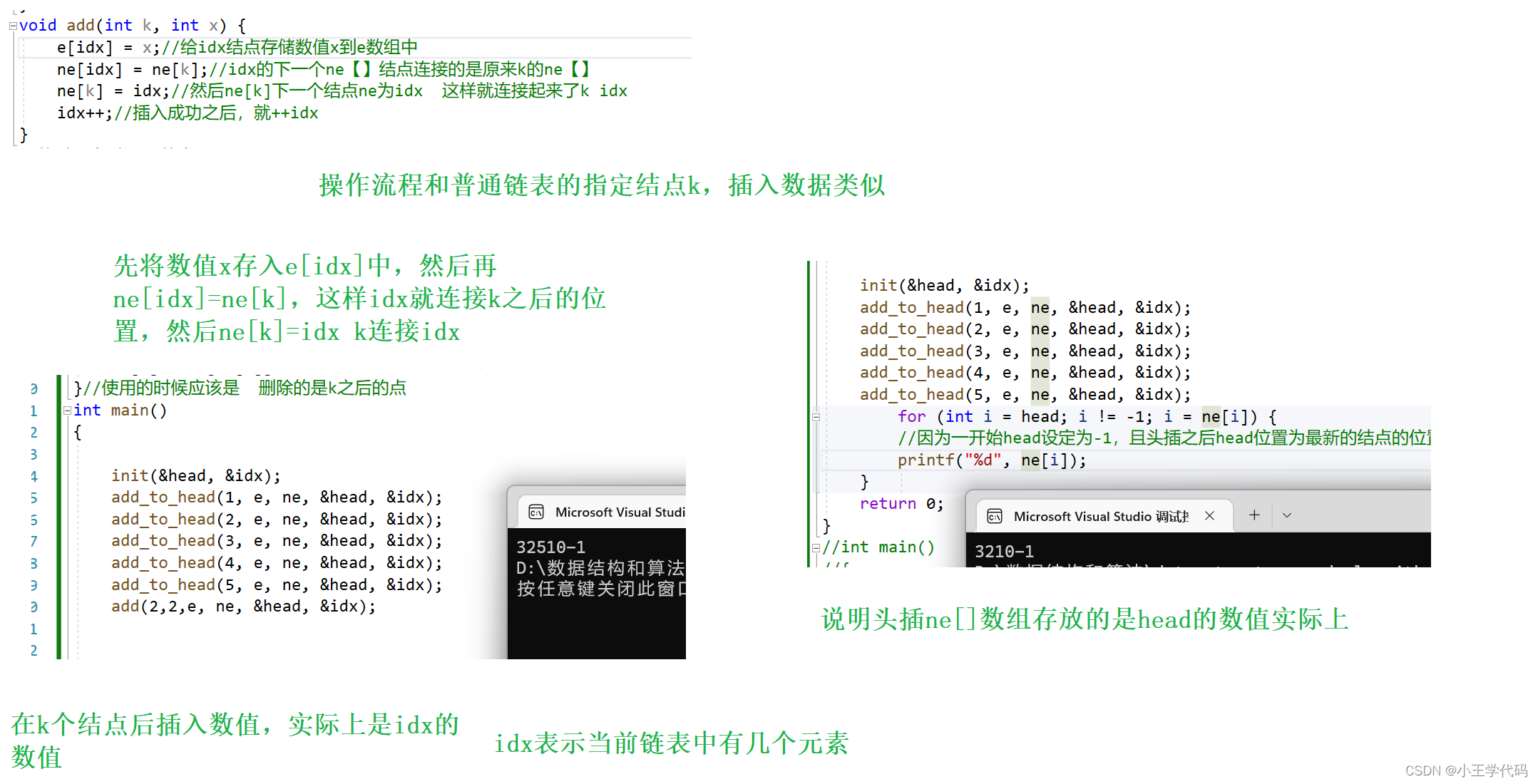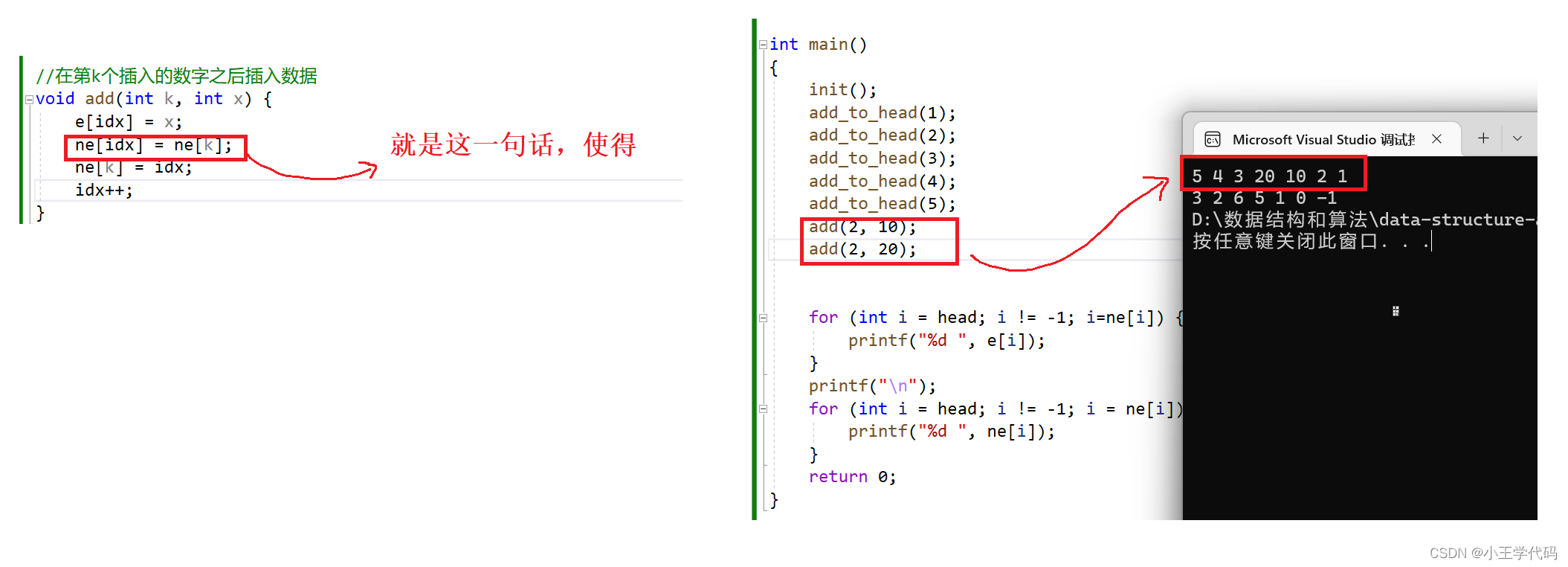# 【数据结构和算法】使用数组的结构实现链表（单向或双向）

1.数组的优缺点

2.链表的优缺点

3.总结

1.认识构造、初始化

2.将x插入到头结点

3.将x插入到第k次插入数值之后的位置

4.删除第k次插入的结点

1.初始化

2.在第k次插入的点的右边插入x

3.删除第k个点

# 前言

``````typedef struct ListNode {
int data;
struct ListNode* next;
}List;``````

``````head	表示头节点
e[N]	表示存储结点数值的数组
ne[N]	表示结点的下一个结点的位置
idx   表示当前存储元素的位置   当前存储到哪里了就是``````

接下来我们来实现单链表，以及双向链表

# 一、用数组结构的好处

1.在搞算法的时候，使用数组的形式去模拟链表，会使得运算速度变快，更加适合写算法，打比赛的小朋友。

2.在笔试的适合，会更快的创建实现链表的基础功能，进行插入删除元素，并根据下标直接找到所需元素等

## 1.数组的优缺点

1.在任意位置删除和插入操作的时候，就会涉及到部分元素的移动，这样的话我们对于数组的任意位置的删除和插入的操作的时间复杂度为O(n)。

1>在i点后面插入数据，那么就需要i+1位置以及之后的元素，整体后移一位（for循环操作），然后再将插入的数据放在i+1的位置上

2>在i点之后删除元素，那么就需要，将i+1以及之后的元素，整体前移一位，总元素个数减一

## 2.链表的优缺点

1.链表也是一种线性结构，但是他存储空间是不连续的（物理不连续，逻辑连续），链表的长度是不确定且支持动态扩展的。每次插入元素的时候，都需要进行申请新节点，然后赋值，插入链表中。

# 二、用数组实现链表

## 1.认识构造、初始化``````//使用c++更简单，先用c++的形式实现
const int N = 100010;
int head, e[N], ne[N], idx; //全局变量
void init() {
idx = 0;//进行初始化的操作，idx为当前链表中（数组）最后一个元素（末尾），下标位置
}
``````

## 2.将x插入到头结点``````void add_to_head(int x) {
e[idx] = x;//将x数值存入到e[]数组中
idx++;
}``````

## 3.将x插入到第k次插入数值之后的位置``````//在第k个插入的数字之后插入数据
void add(int k, int x) {
e[idx] = x;
ne[idx] = ne[k];
ne[k] = idx;
idx++;
}``````

## 4.删除第k次插入的结点

``````//将下标为k的点后面的点删掉
void remove(int k, int ne[]) {
ne[k] = ne[ne[k]];//表示k的下一个位置（ne）为下一个位置的下一个位置，这样跳过了原来的ne[k]结点
}//使用的时候应该是  删除的是k之后的点``````

# 三、完整代码演示

``````#define _CRT_SECURE_NO_WARNINGS
#include<stdio.h>
#include<stdlib.h>

const int N = 100010;
int n, e[N], ne[N], idx, head;

//初始化
void init() {
idx = 0;
}

//头插
e[idx] = x;
idx++;
}

//在第k个插入的数字之后插入数据
void add(int k, int x) {
e[idx] = x;
ne[idx] = ne[k];
ne[k] = idx;
idx++;
}
//删除第k的插入的数据
void remove(int k) {
ne[k] = ne[ne[k]];
}

int main()
{
init();
for (int i = head; i != -1; i=ne[i]) {
printf("%d ", e[i]);
}
printf("\n");
for (int i = head; i != -1; i = ne[i]) {
printf("%d ", ne[i]);
}
return 0;
}

``````

# 四、数组实现双向链表

## 1.初始化

``````//初始化
// e[]表示节点的值，l[]表示节点的左指针，r[]表示节点的右指针，idx表示当前用到了哪个节点
int m;
const int N = 100010;
int e[N], l[N], r[N], idx;
void init() {
//0表示为左端点，1表示为右端点
r = 1;
l = 0;
idx = 2;//从2开始
}``````

e[]表示节点的值，l[]表示节点的左指针，r[]表示节点的右指针，idx表示当前用到了哪个节点

## 2.在第k次插入的点的右边插入x

``````//在第k次插入的点的右边插入x；

void add(int k, int x) {
e[idx] = x;//数值x给当前idx位置的e数组存储
r[idx] = r[k];//将新节点的左右两端分别连接k的后一个结点r[k]和k本身
l[idx] = k;
l[r[k]] = idx;//然后将k的右端点的左端点连接idx
r[k] = idx;//最后将k的右端点连接idx
}
``````

## 3.删除第k个点

``````//删除第k个点
void remove(int k) {
//就是将k的左端点和右端点相互连接
l[r[k]] = l[k];
r[l[k]] = r[k];
}
``````

# 五、完整代码

``````#include<stdio.h>
#include<stdlib.h>
#include<malloc.h>
#include<assert.h>
//使用数组的形式实现双向链表

//e[N]	表示存储结点的数值
//l[N]	表示当前结点的左结点位置
//r[N]	表示当前结点的右节点位置
//idx	表示当前结点存储的位置

//初始化
int m;
const int N = 100010;
int e[N], l[N], r[N], idx;
void init() {
//0表示为左端点，1表示为右端点
r = 1;
l = 0;
idx = 2;//从2开始
}

//在第k次插入的点的右边插入x；

void add(int k, int x) {
e[idx] = x;//数值x给当前idx位置的e数组存储
r[idx] = r[k];//将新节点的左右两端分别连接k的后一个结点r[k]和k本身
l[idx] = k;
l[r[k]] = idx;//然后将k的右端点的左端点连接idx
r[k] = idx;//最后将k的右端点连接idx
}

//删除第k个点
void remove(int k) {
//就是将k的左端点和右端点相互连接
l[r[k]] = l[k];
r[l[k]] = r[k];
}
``````